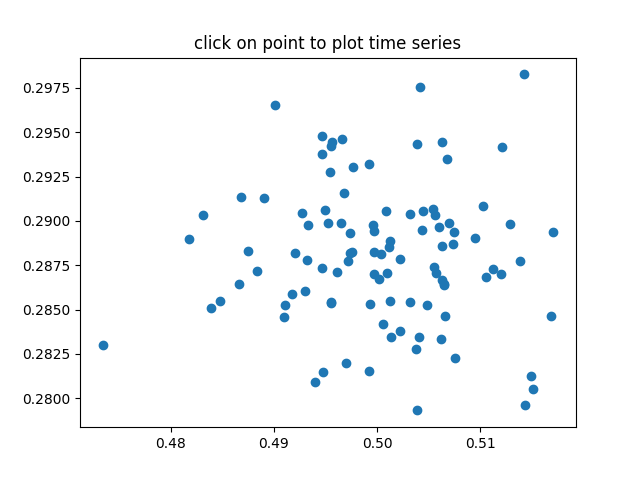# Pick Event Demo2¶

compute the mean and standard deviation (stddev) of 100 data sets and plot mean vs stddev. When you click on one of the mu, sigma points, plot the raw data from the dataset that generated the mean and stddev.import numpy as np
import matplotlib.pyplot as plt

X = np.random.rand(100, 1000)
xs = np.mean(X, axis=1)
ys = np.std(X, axis=1)

fig, ax = plt.subplots()
ax.set_title('click on point to plot time series')
line, = ax.plot(xs, ys, 'o', picker=5)  # 5 points tolerance

def onpick(event):

if event.artist != line:
return True

N = len(event.ind)
if not N:
return True

figi, axs = plt.subplots(N, squeeze=False)
for ax, dataind in zip(axs.flat, event.ind):
ax.plot(X[dataind])
ax.text(.05, .9, 'mu=%1.3f\nsigma=%1.3f' % (xs[dataind], ys[dataind]),
transform=ax.transAxes, va='top')
ax.set_ylim(-0.5, 1.5)
figi.show()
return True

fig.canvas.mpl_connect('pick_event', onpick)

plt.show()


Keywords: matplotlib code example, codex, python plot, pyplot Gallery generated by Sphinx-Gallery